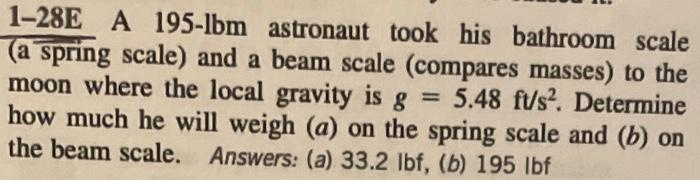Home / Expert Answers / Mechanical Engineering / 1-28e-a-195-lbm-astronaut-took-his-bathroom-scale-a-spring-scale-and-a-beam-scale-compares-masse-pa999

# (Solved): 1-28E A 195-lbm astronaut took his bathroom scale (a spring scale) and a beam scale (compares masse ...1-28E A 195-lbm astronaut took his bathroom scale (a spring scale) and a beam scale (compares masses) to the moon where the local gravity is $$g=5.48 \mathrm{ft} / \mathrm{s}^{2}$$. Determine how much he will weigh $$(a)$$ on the spring scale and $$(b)$$ on the beam scale. Answers: (a) $$33.2 \mathrm{lbf}$$, (b) $$195 \mathrm{lbf}$$

We have an Answer from Expert